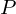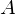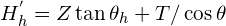# Extraction of the integrated intensities

Extraction of the integrated intensities (%extraction command)

The extraction process is devoted to the decomposition of the measured powder diffraction pattern for extracting the single integrated intensities associated to each reflection in the pattern (Altomare et al., 1995). From the integrated intensities the experimental structure factor moduli are derived. To calculate the integrated intensities, EXPO2014 uses the Le Bail algorithm (Le Bail et al., 1988), suitably combined with a least squares procedure minimizing the quantity:(1)

The summation in (1) goes over all the data points,= observed intensity at the i-th step= calculated intensity at the i-th step(2)

The summation over h includes neighbouring Bragg h reflections,= scale factor= Lorentz – polarization factor= reflection multiplicity= structure factor= profile function= asymmetry function= background intensity at the i-th step.

The background in (2) is automatically estimated and described by the polynomial function (Young, 1993)m, the polynomial degree, is automatically defined by the program as the number which provides the best fit of the background interpolation points.

From the pattern a standard reference peak is automatically selected. The standard peak fitting is performed to provide the starting values of the variables to refine in the least squares procedure.
The full pattern is automatically divided into intervals. The refined parameters derived by the fitting of each interval will be taken into account for the final full pattern fitting.
The following profile shape functions are available for user choice:Pearson VIIGaussianLorentzianPseudo-Voigt

whereThe default choice is the Pearson VII function. In this caseotherwiseThe peak shape, the asymmetry, the cell and the background parameters are all refined by the program.

Directives in the %extraction command

The following directives must be added after the command %extraction in the input file to activate some specific non-default procedures:

all
To activate the procedure of selection of the profile function type (among Pearson VII, Gaussian, Lorentzian, Pseudo-Voigt) from the best fit of each interval.

first
To activate the procedure of selection of the profile function type (among Pearson VII, Gaussian, Lorentzian, Pseudo-Voigt) from the best fit of the first interval.

standard
To activate the procedure of selection of the profile function type (among Pearson VII, Gaussian, Lorentzian, Pseudo-Voigt) from the best fit of the standard peak.

gaussian
To select the gaussian function.

lorentzian
To select the lorentzian function.

pvoigt
To select the Pseudo-Voigt function.

interval n
n is the number of intervals in which the experimental powder pattern is divided (max 8). n is only given if the user wants to change the automatic division of the pattern.

ncycle n
n is the number of cycles for each interval (default value is 60).

nosingle
To omit the preliminary fitting of the standard peak (automatically selected by the program). In this case a preliminary fitting of the first interval is carried out.

lowb
To reduce the background by a constant factor equal to the minimum value  of the observed counts in the experimental pattern.

noba
To skip the background coefficients refinement.

polynomial k
k is the background polynomial degree (max 10). k is given if the user wants to use a fixed degree in each interval and not the degree automatically evaluated by the program.

smoothing
To activate the smoothing procedure described by Brückner (Brückner, 2000) for estimating the background. In this case the background coefficients are not refined.

nocell
To skip the cell parameters refinement.

random p
To activate the random procedure (Altomare et al., 2001) in order to improve the quality of the extracted structure factor moduli values. p is the coefficient for the reflection overlapping definition (default value is 0.5).

frecycle filename.hkl
If the file filename.hkl, containing hkl and the corresponding F-squared values, is available from a previous run of EXPO2014, or from any other source, the Le Bail algorithm starts, for a new decomposition process, by using the provided F-squared values as starting values.

damping x
x is the damping factor used in the least squares routine (default value 0.1). (It is convenient to use a small x value).

width x
x is the factor which, multiplied by the FWHM of each peak in the experimental pattern, gives the total peak width. (In this case the automatic calculation of x is not taken into account).

zeroprofile x
x is the 2θ zero shift value supplied by the user (it is used to correct the 2θ observed positions of the experimental counts). In this case the automatic calculation of the 2θ zero shift is skipped.

slow
To stop the run of EXPO2014 after some relevant calculations and wait for the user graphic interaction to go on. This directive can be used if the graphic interface is active.

verbose
To have a more detailed print in the output file. In this case, the Rp and Rwp values and the values of the refined variables are printed interval by interval.

Examples

Example 1
A Pseudo-Voigt function is used and the background in each interval is described by a constant, the graphic window is suppressed.

 %nowindow
%structure mes
%job MES - data from home diffractometer
%data
pattern mes.pow
cell 8.588 9.931 11.105 90.0 93.754 90.0
content C 24 N 4 O 20 S 4 H 52
spacegroup p 21/c
wavelength 1.5406
%extraction
pvoigt
polynomial 0
%continue

Example 2
The starting values of the integrated intensities in the extraction step by the Le Bail formula are supplied by the user in the file “yono.hkl” and the number of least-square cycles is set to 40.

 %structure yono
%job YONO - Synchrotron data
%data
pattern yono.pow
cell 9.385 16.394 3.627 90.000 101.067 90.000
content Y 8 O 26 N 2 H 18
spacegroup p 21
wavelength 1.2323
synchrotron
%extraction
frecycle yono.hkl
ncycle 40
%continue

Example 3
The preliminary fitting of the standard peak is skipped and the starting 2
θ zero shift value is supplied by the user (the program refines it).

%structure agpz
%job AGPZ - data from home diffractometer
%data
pattern agpz.pow
cell 6.526 20.059 6.464 90.000 90.000 90.000
spacegroup p b c a
content Ag 8 N 16 C 24 H 24
wavelength 1.54056
%extraction
nosing
zeroprof 0.02
%continue
References

Altomare A., Burla M.C., Cascarano G., Giacovazzo C., Guagliardi A., Moliterni A.G.G. and Polidori G. (1995). J. Appl. Cryst. 28, 842-846.
Altomare A. , Giacovazzo C., Moliterni A. G. G. and Rizzi R. (2001). J. Appl. Cryst. 46, 476-482.
Brückner S. (2000). J. Appl. Cryst. 33, 977-979.
Le Bail, A., Duroy, H., Fourquet, J.L. (1988).  Math. Res. Bull. 23, 447-452.